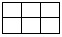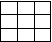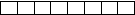# User Forum

Subject :Sam    Class : Sample

Which figure has an area of 8 sq. units and a perimeter of 12 units? (Each square block is of side 1 unit)

ABCDwhere did this (each square block is of side 1 unit) come from.

Class : Class 5

Class : Class 6

Class : Class 6

## Ans 4:

Class : Class 4
( B ) 4 x 2 = 8 4 4 2 2 = 12

Class : Class 5
THANKS

## Ans 6:

Class : Class 5
(Breath*2) (length*2)=perimeterBreath*length=area